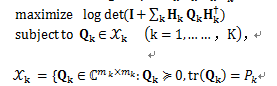# Solution changed when changed a constant parameter in the code

Here is the objective function:（PS:In the above equation Hk is constant matrix and I is the unit matrix.the blackbody means the variable is a matrix ,and the usual is a scalar.)
To simplify, l set the same parameters mk(which represents the users’ antenna number in a system), although actually they may change with the k,The wrong result is when the users up to bigger than 5,then the status is “Failed”.

MATLAB code:
User=6;%the number of users in the system
Nr=8;%the number of rhe BS’s receive antennas
Nt=4;%the number of each user’s antennas
NO=1;%the noise power
Eb=1;%the signal power

cvx_begin

variable Qk_all(Nt,Nt,User)
expression w_all(Nr,Nr,User);
for iUser=1:User
w_all(:,:,iUser)=H(:,:,iUser)*Qk_all(:,:,iUser)*H(:,:,iUser)’;
end

maximize log_det(eye(Nr)+sum(w_all,3))
subject to
for iUser=1:User
trace(Qk_all(:,:,iUser))==1;
Qk=diag(Qk_all(:,:,iUser));
for iAntenna=1:Nt
Qk(iAntenna)>=0;
end
end

cvx_end

Thank you for the help in advance!

What‘s stranger! When I comment this line “expression w_all(Nr,Nt,User)”,Matlab don’t report errors while at first I didn’t write this statement,it gives me a crypic error as following:

??? The following error occurred converting from cvx to double:
Error using ==> double
Conversion to double from cvx is not possible.

I just feel very confused! So can anyone tell me the reason?